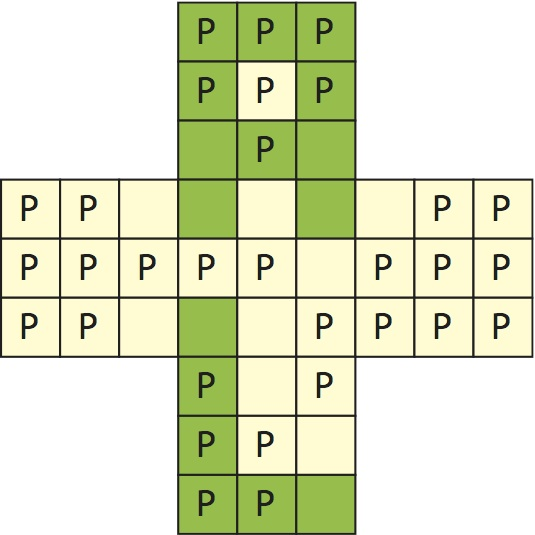Le Monde puzzle [#1650]A penultimate Le Monde mathematical puzzle  before the new competition starts [again!]

For a game opposing 40 players over 12 questions, anyone answering correctly a question gets as reward the number of people who failed to answer. Alice is the single winner: what is her minimal score? In another round, Bob is the only lowest grade: what is his maximum score?

For each player, the score S is the sum δ¹s¹+…+δ⁸s⁸, where the first term is an indicator for a correct answer and the second term is the sum over all other players of their complementary indicator, which can be replaced with the sum over all players since δ¹(1-δ¹)=0. Leading to the vector of scores

worz <- function(ansz){
scor=apply(1-ansz,2,sum)
return(apply(t(ansz)*scor,2,sum))}

Now, running by brute-force a massive number of simulations confirmed my intuition that the minimal winning score is 39, the number of players minus one [achieved by Alice giving a single good answer and the others none at all], while the maximum loosing score appeared to be 34, for which I had much less of an intuition!  I would have rather guessed something in the vicinity of 80 (being half of the answers replied correctly by half of the players)… Indeed, while in SIngapore, I however ran in the wee hours a quick simulated annealing code from this solution and moved to 77.And the 2018 version of Le Monde maths puzzle competition starts today!, for a total of eight double questions, starting with an optimisation problem where the adjacent X table is filled with zeros and ones, trying to optimise (max and min) the number of positive entries [out of 45] for which an even number of neighbours is equal to one. On the represented configuration, green stands for one (16 ones) and P for the positive entries (31 of them). This should be amenable to a R resolution [R solution], by, once again!, simulated annealing. Deadline for the reply on the competition website is next Tuesday, midnight [UTC+1]

This site uses Akismet to reduce spam. Learn how your comment data is processed.